# Transistor

Subjects:

• General
• Health Benefits
• The transistor as a switch
• The transistor as an amplifier
• Transistor characteristic
• Example circuit with a characteristic
• Darlington transistor

General:
Transistors have 2 different applications, they are used as:

• Amplifier (think of an audio amplifier)
• Switch (a transistor can switch large powers very quickly and is used in, among other things, control devices in the car).

Transistors are powered by current. In microprocessors, for example, one wants to keep the current intensity as low as possible in connection with heat development. The MOSFET is often used in this.

There are 2 types of transistors, namely the NPN and the PNP transistor. These are described below.

NPN Transistor:

The B stands for "Base", the C for "Collector" and the E for "Emittor",
With the NPN transistor, the arrow points away from the transistor. This transistor is often used when it is a 'ground circuit', where the Emitter is connected to ground.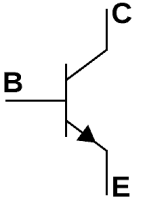PNP transistor:

In the case of the PNP transistor, the arrow points to the transistor. A handy mnemonic for PNP is therefore “Arrow To Plate”.Operation:
In automotive technology, the transistor is most commonly used as a switch, so we'll go into that now. Let's take an NPN transistor as an example.

The image shows the base on the left, above the collector and below the Emitter. When a base current starts flowing (blue arrow), it follows its way to the Emitter. This also causes a collector current to flow to the Emitter. As soon as the base current is lost, the current from collector to Emitter also stops.
If half a base current starts to flow, half the current (compared to I max.) also starts to flow. So it can be clearly seen that the current that is switched by the transistor (from C to E) is completely dependent on the height of B.
A transistor also always has voltage losses due to the PN junction. Between the Base and Emitter it is 0,7 Volt and between the Collector and the Emitter 0,3 Volt.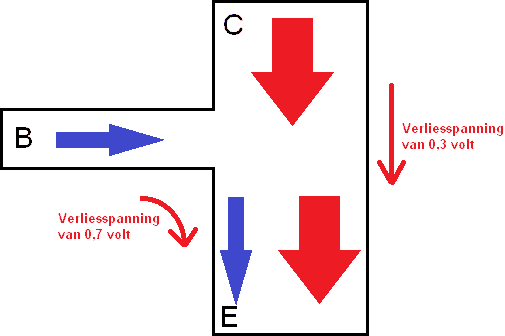The transistor as a switch:
In the following example, a 12 volt / 5 watt lamp is controlled with a transistor. The voltage of UB1 (voltage source 1) is the battery voltage of 12 volts. The lamp is grounded. The base of the transistor is controlled with UB2; “the voltage source 2” of 6 volts.
The loss voltage between Collector – Emitter (UCE) is 0,3 volts, and between Base – Emitter (UBE) 0,7 volts. We will see this in the calculation below. The amplification factor is set at 200. It can always be different. The gain factor is the ratio between the base current and the Collector-Emittor current.

A circuit must always be executed with a certain resistance (in the above diagram RB). If this resistor were not present, the transistor would immediately fail. The value that the resistor RB should have depends on all factors; namely voltages on both UB1 and UB2 and the required current for the components (resistors or lamps), etc. We will now calculate the load resistance RB.To calculate the load resistance RB, first the resistance through the lamp must be calculated.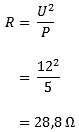Now that the resistance RL is known, the collector current (IC) can be calculated.

UCEsat stands for “saturation”, ie; saturation. As soon as the transistor is conductive, a voltage drop of 0,3 volts occurs between the points C and E (Collector – Emittor).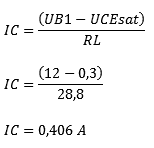The next step is to determine the base current (IB):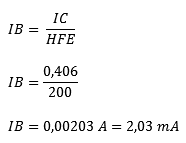A safety margin (IBK) of 1,5 x IB applies to each transistor circuit. So the value of IB has to be multiplied by 1,5 again. The reason for this will be explained later.
The base current is only 12% of the Collector-Emittor current. Now it can be clearly seen that a transistor can be turned into a large main current from a small bass current.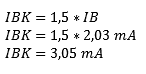Now that all currents in the diagram are known, the resistance RB can be calculated.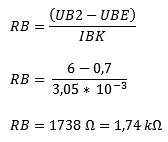UBE is the voltage between the Base and the Emitter. Due to the conductive material in the transistor, there is always a voltage drop of 0,7 Volt between the points B and E.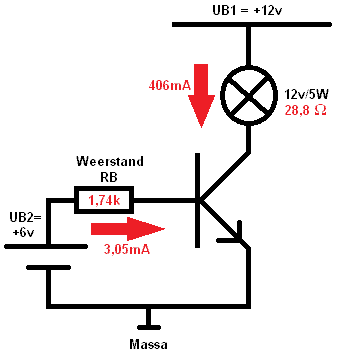There are no standard resistors which are exactly 1,74k (Kilo-Ohm). A standard resistor with a different value must therefore be chosen. The choice must be made from the available resistors from the E12 series.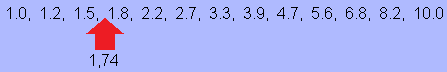The required resistance of 1,74k is between 1,5k and 1,8k. In that case, the lower resistance value should be chosen; for 1,5k. This is so that aging and wear of the components do not affect the currents in the circuit.

The transistor as an amplifier:
The transistor can be used as an amplifier. The base current can be changed by turning a potentiometer. By varying the base current, the gain voltage, and thus the voltage across the collector-emitter, changes.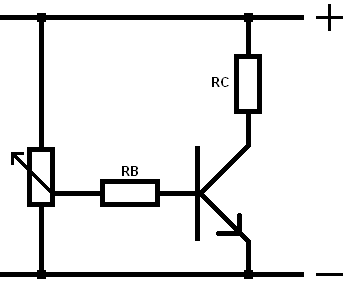Transistor Characteristic:
A characteristic can be made of an NPN transistor, see the image below: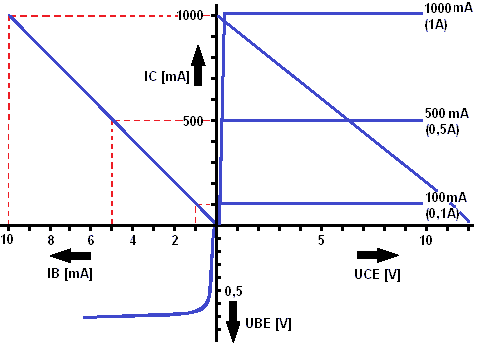1st quadrant (top right) = UCE – IC
Up to 0,3 volts the line slopes upwards. This area is UCEsat (saturation of the transistor). After that, the line runs almost horizontally.

2st quadrant (top left) = IB – IC
Here the relationship between UB and IC is indicated. IC = HFE x IB, with in this characteristic HFE = 10, so IC is 10 times as large as IB. The safety factor of IB = 1,5 x IBK has not yet been taken into account.

3st quadrant (bottom left) = UBE – IB
The loss voltage between the base and Emitter of a transistor is the threshold voltage of a diode. The threshold voltage is 0,7 Volt. From this voltage the transistor starts to conduct and the base current IB starts to flow. This too can be traced back to the characteristic.

Example circuit with a characteristic:
Now it is time for a (simple) example circuit with a corresponding transistor characteristic. Here the IB = 1,5 x IBK is included, resulting in a horizontal line on the axis of IB. In the circuit below, UB1 is the battery voltage and UBE (base-emitter voltage) comes from a switch or signal in a control unit. To calculate the current on UBE, the current IC (collector current) must first be calculated;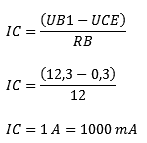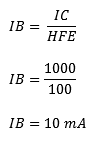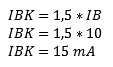Now we know that a current of 15mA must flow through the base of the transistor to make the transistor (with the mentioned UB1 and RB) conduct completely, including the safety factor. Then the characteristic can be filled in: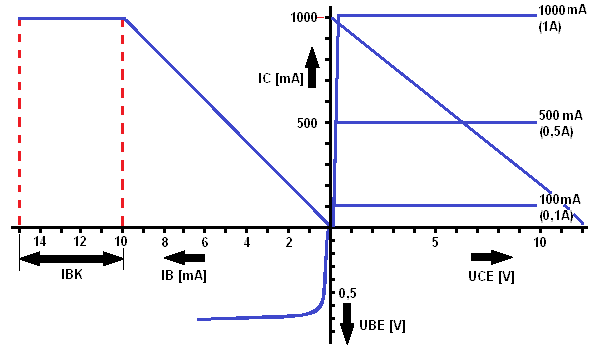In this characteristic it can be seen that the IB (current at the base) increases to 10mA. This part, from 0 to 10 mA, is calculated with the formula: IB = IC : HFE. Then the line runs completely horizontal from 10 to 15mA. This part is the gain of 1,5 (from the calculation of IB = 1,5 x IBK). At a base current of 15mA, a collector current (IC) of 1000mA flows.

Transistors are powered by current. In microprocessors, for example, one wants to keep the current intensity as low as possible in connection with heat development. It often contains the MOSFET applied.

Darlington Transistor:
A control device sends a base current to the transistor. A high switching current is accompanied by heat development in the control unit. A Darlington transistor can be used to switch large currents with as little heat as possible in the control unit. The darlington transistor is made up of two different transistors. The collector-emitter current of T1 provides the base current of T2. This produces a large amplification factor, because the amplification factors of both transistors can be multiplied by each other. A very small base current of T1 (just a tenth of a milliampere) is often sufficient to start conducting.error: Alert: Content is protected !!